# Percent weight of material A100755025Melting temperatureof material ALiquidLiquid and solidMelting temperatureof material BSolid255075100Percent weight of material BFIGURE P2.31Temperature

Question
1 views

Considering an alloy of the two soluble components A and B described by a phase diagram Figure P2.31, determine the masses of the alloy that are in the liquid and solid phases at a given temperature if the total mass of the alloy is 100 g, component B represents 60% of the alloy, 25% of the liquid is component B, and 70% of solid is component B.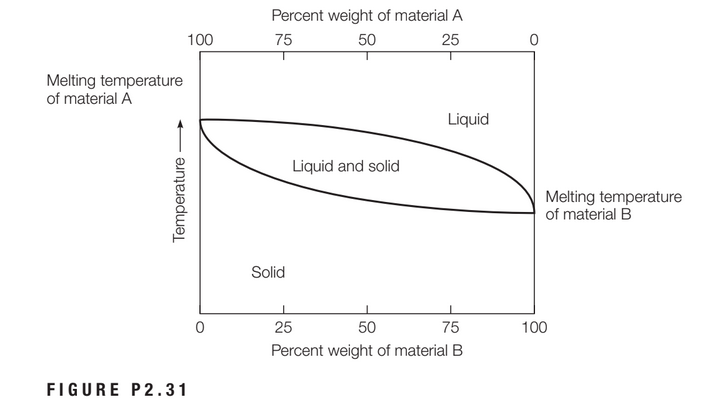help_outlineImage TranscriptionclosePercent weight of material A 100 75 50 25 Melting temperature of material A Liquid Liquid and solid Melting temperature of material B Solid 25 50 75 100 Percent weight of material B FIGURE P2.31 Temperature fullscreen
check_circle

Step 1

Given:

Total mass of the alloy, M = 100 gm

Percentage of component B, PB = 60%

Percentage of component B in liquid, PL = 25%

Percentage of component B in solid, PS = 70%

Step 2

The total mass of the alloy is the sum of the masses of the alloy that are in liquid and solid phase.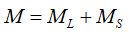Here,

ML = mass of alloy in liquid phase

MS = mass of alloy in solid phase

Substitute the value of total mass in the above equation,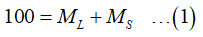The mass of alloy in liquid and solid phase is calculated by the percentages of component B in each phase using the following equation: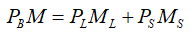Substitute the respective percentages of component B and the total mass of the alloy in the above equation,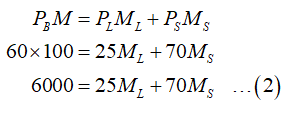From equation (1) and (2),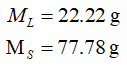...

### Want to see the full answer?

See Solution

#### Want to see this answer and more?

Solutions are written by subject experts who are available 24/7. Questions are typically answered within 1 hour.*

See Solution
*Response times may vary by subject and question.
Tagged in